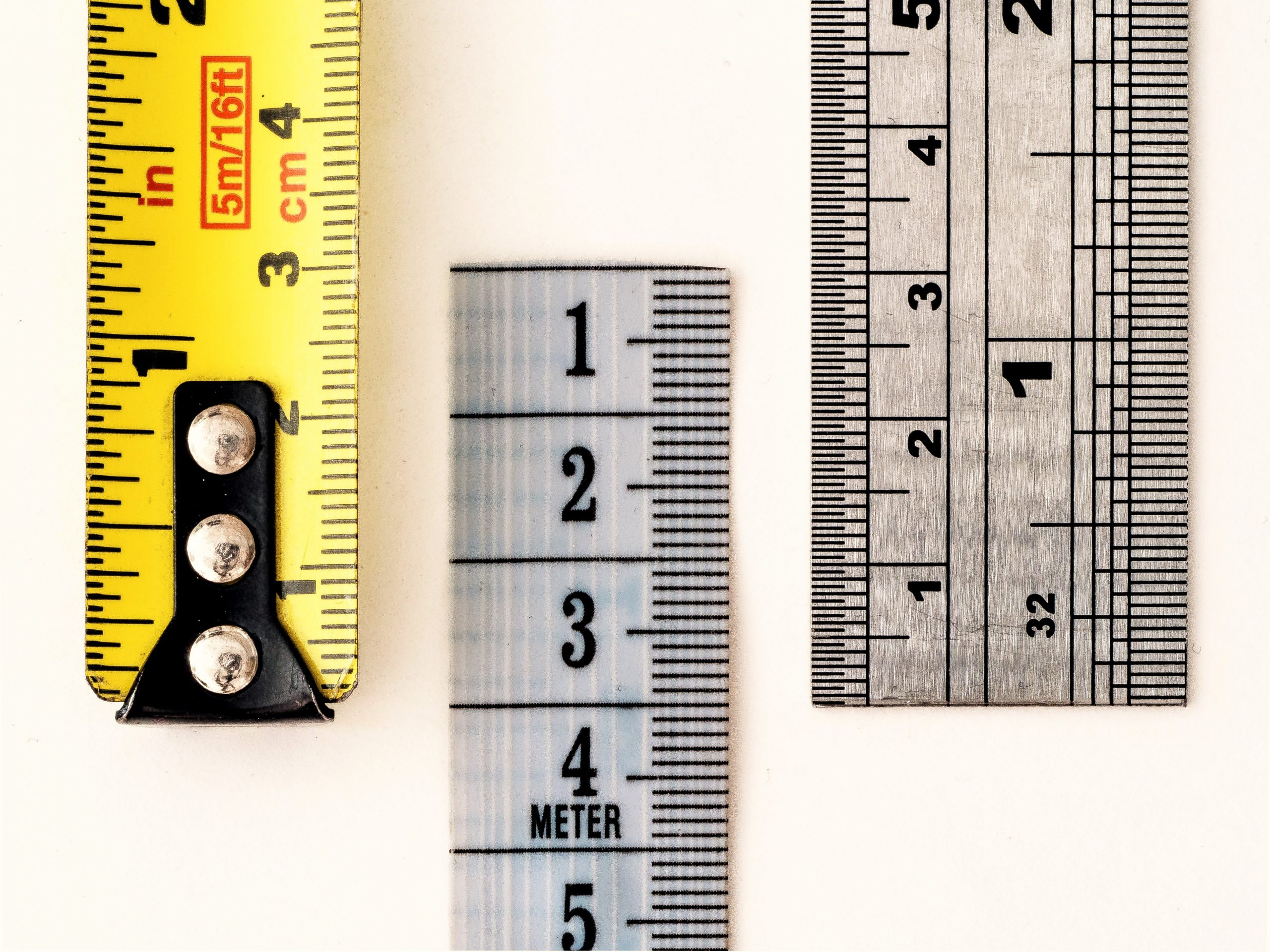# Two plus two equals…?# Time to practice reading numbers.

1 + 1 = 2

2 + 2 = 4

4 + 4 = 8

8 + 8 = 16

16 + 16 = 32

32 + 32 = 64

64 + 64 = 128

128 + 128 = 256

256 + 256 = 512

512 + 512 = 1024

And while we’re at it, here’s a nice little song from a very old movie.

I use to love this one when I was a kid!

In case you’re wondering, an ‘inchworm’ is the baby Geometer Moth.

Because of the way it moves, people think it’s measuring things.